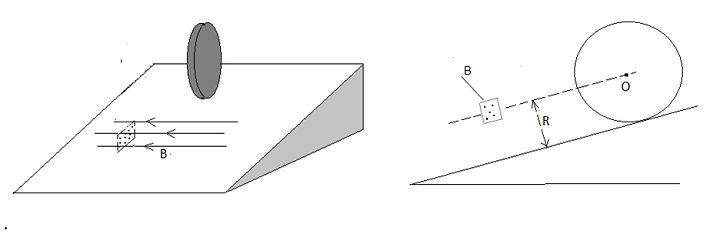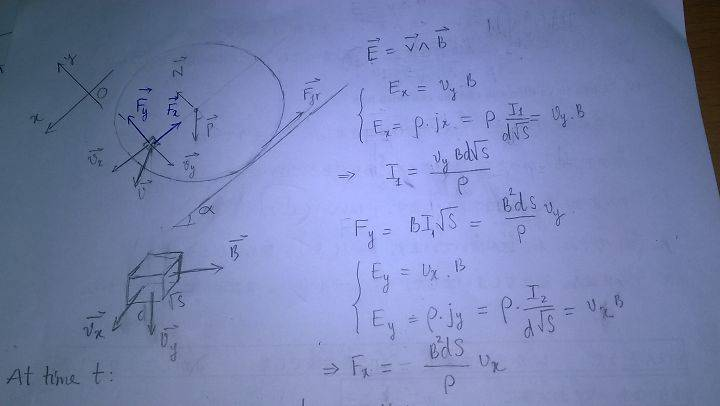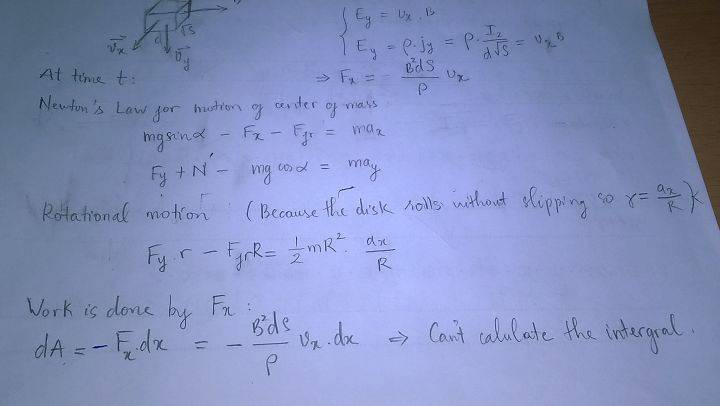# Motion of cylinder

## Homework Statement

At the height h, from the top of inclined plane forming an angle α with the horizontal, a disk slips down which has radius R, thickness d, mass m and resistivity ρ. On inclined plane, an area S is very small square that has a magnetic field B. The magnetic field B is directed horizontally, intensity is constant, at distance R from surface of inclined plane (figure)
Find the velocity of center of mass at time when the disk leaves the magnetic area. Suppose that the disk rolls without slide

## Homework Equations

Newton's law: F=ma $$\vec{E}=\vec{v}×\vec{B}$$
Current density $$\vec{j}=\frac{I(\vec{l}\vec{n})}{S}\vec{n}$$ with \vec{l} is vector unit alongs electric current
Differential form of Ohm's law: $$\vec{E}=ρ\vec{j}$$

## The Attempt at a Solution

Force is appeared by magnetic field, along x-axis and y-axis are relational. Althought I can write Newton's law but I cant find the velocityLast edited:

This looks like an awesome problem.

Since the ball rolls without slipping, what can you say about its total kinetic energy before it goes through the magnetic field, as it goes through the magnetic field, and after it goes through the magnetic field?

Last edited:
I thinked that using kinetic energy theorem. ΔW=ΣA
But I cant find the work of the x-axis is create by Fx (electromagnetism force). Because while disk rolls, the area S moves too. And size of velocity of area S isnt constant. It is relational with the velocity of disk. So equations differential is very difficult.

Delta2
Homework Helper
Gold Member
It seems hard to model the interaction between the magnetic field and the rolling cylinder in terms of forces.

Instead use conservation of energy: I would first use the equations you present in OP to determine the current density ##J=\frac{E}{\rho}## and the current ##I=JS=\frac{Bv}{\rho}S## that is induced, then calculate properly the power dissipated as ##P=I^2R## where ##R=\rho\frac{\sqrt{S}}{d\sqrt{S}}## and assume that this power comes from reducing the kinetic energy (translational+rotational) of the cylinder.

•Hamal_Arietis
We must find the loss enery by heat. ##Q=I^2Rt## , not P. So we must find t, it seems very hard. Your I isnt correct. ##I=\frac{B\sqrt{S}d}{\rho}v##. And this v is velocity of area S in laboratory reference frame. and it changes when the time changes. Thanks your good idea. After I use force to find work. There are the same result. And it cant find the work :(

Last edited:
Delta2
Homework Helper
Gold Member

Write down the expression for the total mechanical energy ##E=E(h(t),v(t))## involving the term ##mgh(t)## and the translational and rotational kinetic energy terms that depend on ##v(t)## and the radius of the cylinder R (we assume the cylinder keeps rolling without slipping through the whole phase).

Then we ll have the differential equation ##I^2R=-\frac{dE}{dt}##.

Last edited:
the I have v(t) but that is a velocity of area S. ##\vec{v}=-\vec{\omega}X\vec{r}+ \vec{v'}## Moever dE= f(h(t);v '(t)) . dE/dt => will appear a(t) . The differential equation seem hard

Delta2
Homework Helper
Gold Member
Ah yes I didn't notice that the velocity of the area S will vary also from ##\omega(t)R## (as the area S enters the cylinder) to 0 (as the area passes through the center of the disk) and then to ##-\omega(t)R## when the area leaves the cylinder.

Either we 'll do an approximation and assume that it remains "constant" ##\omega(t)R## or otherwise the differential equation will be very very hard.

•Hamal_Arietis
haruspex
Homework Helper
Gold Member
Ah yes I didn't notice that the velocity of the area S will vary also from ##\omega(t)R## (as the area S enters the cylinder) to 0 (as the area passes through the center of the disk) and then to ##-\omega(t)R## when the area leaves the cylinder.

Either we 'll do an approximation and assume that it remains "constant" ##\omega(t)R## or otherwise the differential equation will be very very hard.
This is not an area I know anything about, so I may be completely off here. When a conducting plate passes across a magnetic field for some distance does the total work done depend on the speed of the plate? If not, you just need to compute the path length.
I tried searching for equations on this, but could only find an equation for alternating fields.

•Hamal_Arietis
Delta2
Homework Helper
Gold Member
This is not an area I know anything about, so I may be completely off here. When a conducting plate passes across a magnetic field for some distance does the total work done depend on the speed of the plate? If not, you just need to compute the path length.
I tried searching for equations on this, but could only find an equation for alternating fields.

Magnetic field cannot do work on matter, and there are some threads in physics forums about this. However magnetic field creates an electric field which does work on matter and the electric field generated in this case depends on the magnetic field and on the velocity of the surface S ##\vec{v}## , it is ##\vec{E}=\vec{v}\times \vec{B}##.

This problem involves a few approximations
1) We neglect the displacement current term ##dE/dt## which will create an additional magnetic field according to Maxwell-Ampere Law. But ok this is the most usual approximation in these kind of problems

2) We neglect the self induction term. The current density ##\vec{J}=\sigma\vec{E}## that will be generated will create additional magnetic field again according to Maxwell-Ampere Law.

3) In the velocity of the surface S, ##v##, it is ##v=\vec{\omega(t)}\times\vec{R(t)}+\vec{v'(t)}## where ##v'(t)## the translational velocity of the cylinder which is ##\omega(t)R## since the cylinder is rolling without slipping. Seems we must neglect the term ##\vec{\omega(t)}\times\vec{R(t)}## otherwise the differential equation will have additional complexity cause because ##R(t)=R-\int v'(t)dt##. This R(t) varies from +R to -R thus has an average value of 0, maybe is a good idea to neglect it..

•Hamal_Arietis
today, that is what I can doIs forum in Australia or America ?

Last edited by a moderator:
Magnetic field cannot do work on matter, and there are some threads in physics forums about this. However magnetic field creates an electric field which does work on matter and the electric field generated in this case depends on the magnetic field and on the velocity of the surface S ##\vec{v}## , it is ##\vec{E}=\vec{v}\times \vec{B}##.

.
E is just a virtual field, not real. I argree with you that magnetic field cannot do work on matter. And work is done by visual E equals the loss enery by heat

Delta2
Homework Helper
Gold Member
Ok I see you preferred to work with forces, I ll check it and reply tomorrow , I got to go now.

Nidum
Gold Member
Is this problem based on Faraday's disk ?

haruspex
Homework Helper
Gold Member
today, that is what I can doIs forum in Australia or America ?
Ok, so the retarding force is some constant k times the normal velocity. That's what I needed to know.
If x is the displacement of the disc down the slope, with x=-r representing the point at which the leading edge reaches the field, I get
##I\ddot x=A-k\dot x(x^2+r^2)##, for some constants A and I.
We can integrate that directly, or convert to the time-independent form using ##\dot v= v dv/dx##, but neither produces anything I can see how to solve.

Last edited by a moderator:
•Hamal_Arietis
Delta2
Homework Helper
Gold Member
E is just a virtual field, not real. I argree with you that magnetic field cannot do work on matter. And work is done by visual E equals the loss enery by heat

I sense a problem with your working

1) If the electric field is virtual, then the current density (which for sure is real) is solely due to the Lorentz force ##q(v\times B)##. BUT then the Lorentz force can NOT be responsible for two things simultaneously: for generating the current density, and for generating the laplace force ##BI\sqrt{S}## on the current density.

2) If the electric field is real, then where does it come from since there is no term ##\frac{\partial B}{\partial t}##. I suppose it comes from separation of charges , with a mechanism similar that happens in a conductor that has velocity v inside a magnetic field B and has real voltage ##E=Bvl## in its two edges. I believe the top and left side of the square area S will accumulate positive charges, while the bottom and right side of the area S will accumulate negative charges. There will be current due to that voltage difference that will span across the rest portion of the cylinder (the area of the circle disk minus the area S). But I believe the current will be quite different from what you calculate in your workings.

•Hamal_Arietis
Delta2
Homework Helper
Gold Member
I have to ask this, is this problem found in a book, or is it a problem you made yourself Hamal_Arietis?

Cause it just seems hard to model current density (at least the way I view it, with the area S acting as EMF source and the current density in the whole cylinder) hard to model the differential equation, and without exact solution for the differential equation...

It is the exam for gifted student in my country. And the solutions in all exam has been keeped secret since 2010. Yesterday I tried to find ##-\frac{dE}{dt}## but that appeared a(t) and I cant contrinous.
I think we need to stop this problem.

Delta2
Homework Helper
Gold Member
Ok , when you learn the solution please post here, I am almost sure what we missing is some sort of approximation/simplification that simplifies the problem and unlocks the solution.

haruspex
Homework Helper
Gold Member
Ok , when you learn the solution please post here, I am almost sure what we missing is some sort of approximation/simplification that simplifies the problem and unlocks the solution.
Do you agree with Hamal's expression for the retarding force in post #11: F=B2dSv/ρ, where F acts oppositely to the relative velocity v?
If so, you can obtain the differential equation in my post #15. The constants k, A, I are all known. It may be necessary to make an approximation to solve it, though.

Delta2
Homework Helper
Gold Member
Do you agree with Hamal's expression for the retarding force in post #11: F=B2dSv/ρ, where F acts oppositely to the relative velocity v?
If so, you can obtain the differential equation in my post #15. The constants k, A, I are all known. It may be necessary to make an approximation to solve it, though.

Nope I don't agree with that expression and the reason is point (1) post #16. But I might be wrong, if the expression for the force is correct then seems to me your post is correct also.

•Hamal_Arietis
vanhees71
Gold Member
2021 Award
That's a tough problem, and it's not completely clear to me what's given. First of all is the disk slipping or rolling (from the text it says it's slipping), and I guess one should neglect friction.

Qualitatively what happens when the disk, supposed it's a conductor, enters the magnetic field due to the Lorentz force on the conduction charges (in metals electrons) you get a charge separation and an electric field builds up, and this electric field causes a current and dissipation through the resistance of the conductor. So you have to calculate this energy loss. It's not totally clear to me yet, how to do that with the full dynamics, but perhaps it's a good approximation to assume that the Hall voltage and also the velocity of the disc is constant during it is within the magnetic field with the argument that the region where the magnetic field is different from 0 is small.

•Delta2
haruspex
Homework Helper
Gold Member
(from the text it says it's slipping), and I guess one should neglect friction.
But it finishes
Suppose that the disk rolls without slide

Delta2
Homework Helper
Gold Member
Ok well here is my new take of the problem

There will be two Hall Voltages in the square area S.
##E_y=Bv_x\sqrt{S}##
##E_x=Bv_y\sqrt{S}##

Those will create two currents ##I_y## and ##I_x## that will flow throw the whole cylinder but since the rest of the cylinder is not in a magnetic field, we only need consider the laplace force as those currents flow through the area S. it will be

##I_y=\frac{E_y}{R_y}##
##I_x=\frac{E_x}{R_x}##

and the Laplace forces will be

##F_x=BI_y\sqrt{S}##
##F_y=BI_x\sqrt{S}##

those are very similar to what Hamal calculated, they just different up to constants (AND ofcourse the underlying physical reality is totally different, cause we have a real electric field and a real voltage that creates these currents).

So the solution will be similar to what Harupex post, it will just differ to the constants of the differential equation

R_y and R_x are the total resistances of the cylinder that the E_y and E_x voltages "see". I believe they are different but it seems hard for me to calculate em atm. They will be constants though (unless we want to consider effects like skin effect which I am sure we don't)..

Delta2
Homework Helper
Gold Member
Ok, so the retarding force is some constant k times the normal velocity. That's what I needed to know.
If x is the displacement of the disc down the slope, with x=-r representing the point at which the leading edge reaches the field, I get
##I\ddot x=A-k\dot x(x^2+r^2)##, for some constants A and I.
We can integrate that directly, or convert to the time-independent form using ##\dot v= v dv/dx##, but neither produces anything I can see how to solve.

I seem to get a slightly different differential equation ##\alpha\ddot x=\beta-\gamma\dot x ((x-R)^2+\delta)##

haruspex
Homework Helper
Gold Member
I seem to get a slightly different differential equation ##\alpha\ddot x=\beta-\gamma\dot x ((x-R)^2+\delta)##
I took moments about the point of contact (so I=3mr2/2). If the retarding force is F its moment is ##F\sqrt{x^2+r^2}##. The linear velocity of the disc centre is ##\dot x##, so the angular velocity is ##\dot x/r##, and the velocity of the patch of disc in the magnetic field is ##\frac {\dot x}{r}\sqrt{x^2+r^2}##. Thus the moment of F is ##k\frac{\dot x}r(x^2+r^2)##.

Delta2
Homework Helper
Gold Member
There are two forces, ##F_x## and ##F_y##, do you take both force's moments? Also don't understand the expression for moment , is r the radius of disk R or it is something else?

Mine equation is from ##(\sum{F})_x=m\ddot x## where I replace the friction force using the equation of rotational motion that Hamal gives in his hand writing.

haruspex
Homework Helper
Gold Member
There are two forces, ##F_x## and ##F_y##, do you take both force's moments? Also don't understand the expression for moment , is r the radius of disk R or it is something else?

Mine equation is from ##(\sum{F})_x=m\ddot x## where I replace the friction force using the equation of rotational motion that Hamal gives in his hand writing.
r is the radius of the disk. I assumed the retarding force directly opposes the motion of the patch of disk in the field. I.e. if the patch has velocity ##\vec v## then the force is ##\vec F=-k\vec v## for some positive constant k.
If you draw a line from the point of contact of disk with plane to the patch, it will have length ##\sqrt{x^2+r^2}## and be normal to the force.

Last edited:
Delta2
Homework Helper
Gold Member
r is the radius of the disk. I assumed the retarding force directly opposes the motion of the patch of disk in the field. I.e. if the patch has velocity ##\vec v## then the force is ##\vec F=-k\vec v## for some positive constant k.
If you draw a line from the point of contact of disk with plane to the patch, it will have length ##\sqrt{x^2+r^2}## and be normal to the force.
1) Seems to me the correct expression for the arm of the moment is ##\sqrt{(R-x)^2+R^2}##. I consider t=0,x=0 when the area S first enters the disk from the left. As x increases from 0 to 2R (R-x) varies from R to -R which I believe is the correct thing here...
2) How do you know that the total force ##\vec F=-k\vec v## is always perpendicular to the arm? (sorry if my geometry skill fails me here and I don't see what it might be obvious)..

Last edited:
haruspex
•